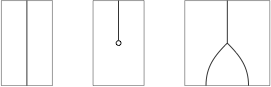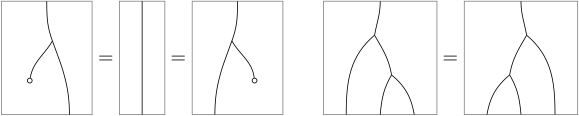# understanding programming monads through diagrams

I am trying to understand better how the category definition of monad is related to the computer science definition. nlab has a rather terse definition of Monad in terms of a bicategory.

• an object $a$
• an endomorphism $t: a \to a$
• $2$-cells $\mu, \eta$ (multiplication and unit).

For the case of programming languages, what are the objects (0-cells), morphisms (1-cells) and 2-morphisms (2-cells)? I am guessing the object is a type and multiplication is the >>= operator (some way of putting commands in succession). I won' t know what the endomorphism $t$ could be.

I was told that list is a monad, so is maybe and IO but I don't see the category or endomorphisms there.

Alternatively they have another definition that kind of makes sense:

Indeed, one can define a monad on an object a of a bicategory K as just a monoid in the endomorphism category K(a,a).

Are the endomorphism the lines of code preserving the type a?

nLab goes on to say, that monads can be represented as string diagrams. Here areThe axioms for a monad - could be represented in terms of the >>= operator in a language like Haskell - are represented in terms of string diagrams.Then, can a computer program be represented in terms of these diagrams?

This is a sequel to a previous question: https://cs.stackexchange.com/questions/30757/how-is-io-a-monad and can be migrated at the discretion of the admins.

I think the nLab definition is too abstract and is confusing you more than anything. Perhaps you should start from Wikipedia? Anyway, here are some comments.

For the case of programming languages, what are the objects (0-cells), morphisms (1-cells) and 2-morphisms (2-cells)?

In the case of programming languages (and in most usual cases) the bicategory in question is $\mathbf{Cat}$, the 2-category whose 0-cells are categories, 1-cells functors and 2-cells natural transformations.

Spelling out the nLab definition, the object is a category, the endomorphism is an endofunctor and multiplication and unit are natural transformations.

A typed functional programming language usually induces a category as follows: the objects of the category are types; a morphism $A\rightarrow B$ is an expression (i.e., a program) of type B containing a parameter of typa A, modulo evaluation. I am not overly familiar with Haskell but I believe that people call the induced category $\mathbf{Hask}$, so a Haskell monad is nothing but an endofunctor (with suitable natural transformations) of $\mathbf{Hask}$. If I am not mistaken, in that context the unit is called "return", whereas multiplication is called "bind", i.e., the >>= operator (the category-theorist's definition of multiplication $\mu$ may be easily recovered from >>=, and vice versa).

Are the endomorphism the lines of code preserving the type a?

No. As I said above, the endomorphisms are endofunctors. For Haskell, the object a is not a type but the category $\mathbf{Hask}$.

Then, can a computer program be represented in terms of these diagrams?

The answer is actually yes, but not through the connection you are describing. In fact, the string diagrams you mentioned live in the 2-category $\mathbf{Cat}$, whereas programs live in the category induced by the programming language. A little more on this topic may be found in the answers to this cstheory.SE question.

• Is it possible to illustrate the equivalence of the diagrams using code rather than just category theory? Oct 10, 2014 at 18:43
• Yes of course! The Wikipedia article on monads does this precisely using Haskell, with respect to the bind/return formulation of monads. The nLab diagrams correspond to the multiplication/unit formulation, but in that case too may be formulated using code. In fact, @jozefg already did it in the answer to your previous question. Oct 11, 2014 at 8:11